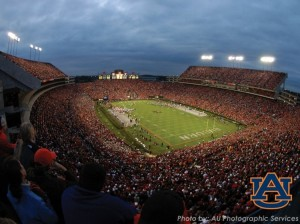Dr. Kent McCorkle, or "Dr. Kent' to his student, has helped thousands of students be successful in chemistry.

# Chemistry Lesson: Structure of the Atom – Protons, Neutrons & Electrons

## Basic Structure of the atom

The atom is very tiny, having a diameter of about 10-10 meters. Keep in mind that’s like saying 0.0000000001 meters, or about one-billionth of a meter across! But within this already extremely small atom is an even smaller region called the nucleus. The nucleus has a diameter of 10-14 m, so it is about 10,000 times smaller than the volume of the atom as a whole. One analogy is to imagine the atom is the size of a football stadium. In that gigantic stadium the nucleus would only be the size of a small marble sitting on the 50-yard line!If this were the volume of the atom… then this would be the volume of the nucleus.

## Subatomic Particles

Within the atom are three basic subatomic particles: protons (p+), neutrons (n0), and electrons (e). We abbreviate the proton as p+ because it has a positive charge, the neutron as n0 because it is neutral, and the electron as e because it has a negative charge.

 Mass (kg) Mass (amu) Charge proton 1.67262×10-27 1.0073 1+ neutron 1.67493×10-27 1.0087 0 electron 0.00091×10-27 0.00055 1-

The protons and neutrons live in the nucleus, while the electrons are orbiting the nucleus similar to the way planets orbit a star.  (We’ll see in a later video the various orbits these electrons can occupy.) In a neutral atom, the number of positively-charge protons must equal the number of negatively-charged electrons. If there were more protons than electrons, then it would have a positive charge overall. While if it had more electrons than protons, it would have an overall negative charge. Atoms with positive or negative charges are called ions. But for atoms they are always neutral and the number of protons equals the number of electrons.

In terms of mass, we see that protons and neutrons are very similar with both having a mass of approximately 1 amu (atomic mass units). Now compare that to the mass of the electrons which are about 1,800 times less massive. As an analogy, if protons and neutrons had a mass around that of a baseball, the mass of an electron would about that of a single grain of rice!If protons and neutrons had the mass of baseballs… then electons would have the mass of a grain of rice.

But where do the massive protons and electrons live? In the tiny nucleus. This means that rather than the mass being spread out evenly across the atom, 99.9% of the mass of an atom is contained in just 1/10,000th of its volume. Going back to the football stadium analogy, it’s as if 99.9% of the mass of that huge stadium was condensed down in to the tiny marble sitting on the 50-yard line. Because so much mass is contained in such a tiny volume this makes the nucleus incredibly dense! For comparison, let’s look at a few other substances that are commonly thought of as dense.

 Density (g/cm3) Lead 11.34 Gold 19.30 Osmium 22.59 Nucleus 1013-1014

Lead is very dense which is why it is often used to block radiation. Its density is 11.34 g/cm3. This means that if you had a cube that was 1 cm x 1 cm x 1cm it would have a mass of 11.34 g. By comparison, the same sized cube of gold would weigh 19.30 g. So a cube of gold would be much heavier than the same sized cube of lead. The most dense element we know of is osmium whose density is 22.59 g/cm3. This means that a 1 cm x 1 cm x 1 cm cube of osmium would be heavier than gold and about twice as heavy as a cube of lead.

But all of these densities pale in comparison to the incredibly dense nucleus. By compressing 99.9% of the mass of the atom in to just 1/10,000th of the volume the density of a typical nucleus is between 10-100,000,000,000,000 g/cm3! So the same 1 cm x 1 cm x 1 cm cube would have a mass between ten and one hundred trillion grams! We cannot fathom how dense this truly is. In fact, if you were able to collect enough nuclei to fill a small matchbox it would have a mass of over 2.5 billion tons! Think about that for a second: this tiny matchbox would have a mass equal to that of a billion cars!= one billion cars